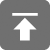# 工学1号馆

home

## 递归与尾递归（C语言）

By Wu Yudong on June 27, 2015

(1) 递归就是在过程或函数里调用自身；

(2) 在使用递归策略时，必须有一个明确的递归结束条件，称为递归出口。

### 基本递归F(4)=4×F(3)　　　　　　　　　　　　递归阶段

F(3)=3×F(2)

F(2)=2×F(1)

F(1)=1　　终止条件

F(2)=(2)×(1)　　　 回归阶段

F(3)=(3)×(2)

F(4)=(4)×(6)

24　　　　　　　　　　　　　　　　  递归完成

int fact(int n) {
if(n < 0)
return 0;
else if (n == 0 || n == 1)
return 1;
else
return n * fact(n - 1);
}

BSS段:（bss segment）通常是指用来存放程序中未初始化的全局变量的一块内存区域。BSS是英文Block Started by Symbol的简称。BSS段属于静态内存分配。#include <stdio.h>
int g1=0, g2=0, g3=0;
int max(int i)
{
int m1 = 0, m2, m3 = 0, *p_max;
static n1_max = 0, n2_max, n3_max = 0;
p_max = (int*)malloc(10);
printf("打印max程序地址\n");
printf("in max: 0x%08x\n\n",max);
printf("打印max传入参数地址\n");
printf("in max: 0x%08x\n\n",&i);
printf("打印max函数中静态变量地址\n");
printf("0x%08x\n",&n1_max); //打印各本地变量的内存地址
printf("0x%08x\n",&n2_max);
printf("0x%08x\n\n",&n3_max);
printf("打印max函数中局部变量地址\n");
printf("0x%08x\n",&m1); //打印各本地变量的内存地址
printf("0x%08x\n",&m2);
printf("0x%08x\n\n",&m3);
printf("打印max函数中malloc分配地址\n");
printf("0x%08x\n\n",p_max); //打印各本地变量的内存地址
if(i) return 1;
else return 0;
}
int main(int argc, char **argv)
{
static int s1=0, s2, s3=0;
int v1=0, v2, v3=0;
int *p;
p = (int*)malloc(10);
printf("打印各全局变量(已初始化)的内存地址\n");
printf("0x%08x\n",&g1); //打印各全局变量的内存地址
printf("0x%08x\n",&g2);
printf("0x%08x\n\n",&g3);
printf("======================\n");
printf("打印程序初始程序main地址\n");
printf("main: 0x%08x\n\n", main);
printf("打印主参地址\n");
printf("argv: 0x%08x\n\n",argv);
printf("打印各静态变量的内存地址\n");
printf("0x%08x\n",&s1); //打印各静态变量的内存地址
printf("0x%08x\n",&s2);
printf("0x%08x\n\n",&s3);
printf("打印各局部变量的内存地址\n");
printf("0x%08x\n",&v1); //打印各本地变量的内存地址
printf("0x%08x\n",&v2);
printf("0x%08x\n\n",&v3);
printf("打印malloc分配的堆地址\n");
printf("malloc: 0x%08x\n\n",p);
printf("======================\n");
max(v1);
printf("======================\n");
printf("打印子函数起始地址\n");
printf("max: 0x%08x\n\n",max);
return 0;
}

### 尾递归

int facttail(int n, int a)
{
if (n < 0)
return 0;
else if (n == 0)
return 1;
else if (n == 1)
return a;
else
return facttail(n - 1, n * a);
}